Publicité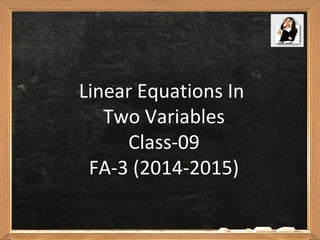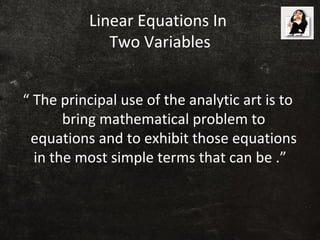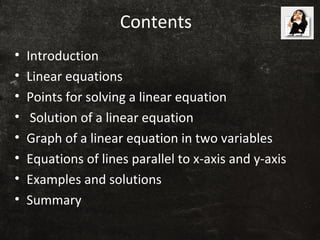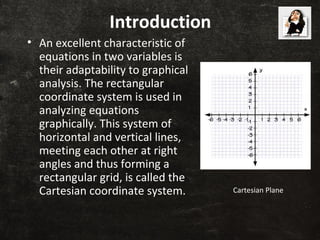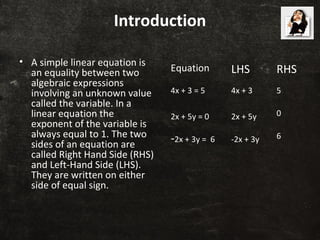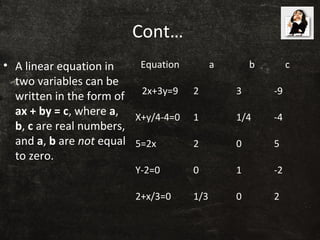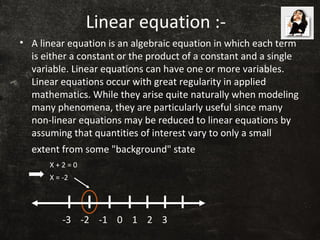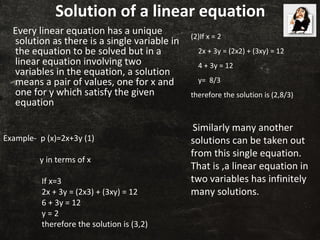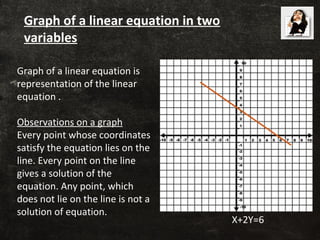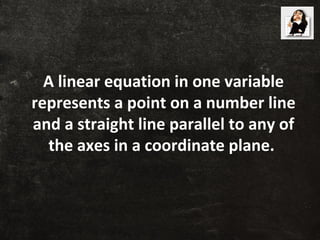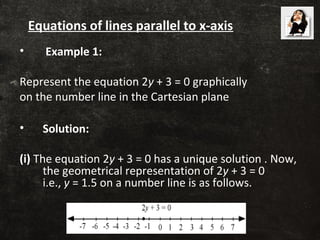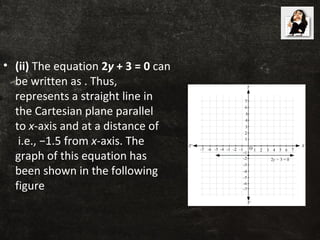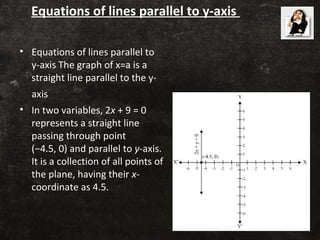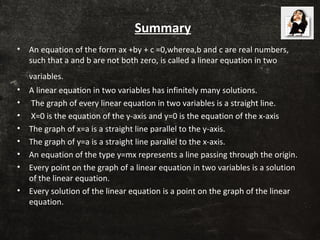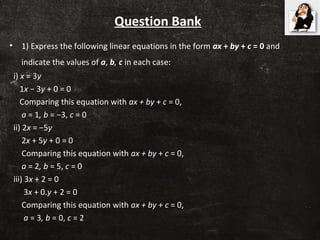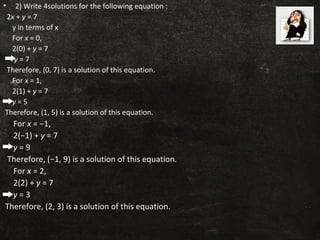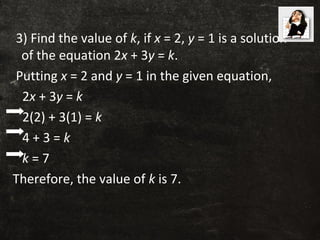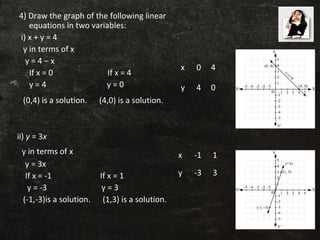1 sur 19
Publicité

### CLASS 9 LINEAR EQUATIONS IN TWO VARIABLES PPT

1. Linear Equations In Two Variables Class-09 FA-3 (2014-2015)
2. Linear Equations In Two Variables “ The principal use of the analytic art is to bring mathematical problem to equations and to exhibit those equations in the most simple terms that can be .”
3. Contents • Introduction • Linear equations • Points for solving a linear equation • Solution of a linear equation • Graph of a linear equation in two variables • Equations of lines parallel to x-axis and y-axis • Examples and solutions • Summary
4. Introduction • An excellent characteristic of equations in two variables is their adaptability to graphical analysis. The rectangular coordinate system is used in analyzing equations graphically. This system of horizontal and vertical lines, meeting each other at right angles and thus forming a rectangular grid, is called the Cartesian coordinate system. Cartesian Plane
5. Introduction • A simple linear equation is an equality between two algebraic expressions involving an unknown value called the variable. In a linear equation the exponent of the variable is always equal to 1. The two sides of an equation are called Right Hand Side (RHS) and Left-Hand Side (LHS). They are written on either side of equal sign. Equation LHS RHS 4x + 3 = 5 4x + 3 5 2x + 5y = 0 2x + 5y 0 -2x + 3y = 6 -2x + 3y 6
6. Cont… • A linear equation in two variables can be written in the form of ax + by = c, where a, b, c are real numbers, and a, b are not equal to zero. Equation a b c 2x+3y=9 2 3 -9 X+y/4-4=0 1 1/4 -4 5=2x 2 0 5 Y-2=0 0 1 -2 2+x/3=0 1/3 0 2
7. Linear equation :- • A linear equation is an algebraic equation in which each term is either a constant or the product of a constant and a single variable. Linear equations can have one or more variables. Linear equations occur with great regularity in applied mathematics. While they arise quite naturally when modeling many phenomena, they are particularly useful since many non-linear equations may be reduced to linear equations by assuming that quantities of interest vary to only a small extent from some "background" state -3 -2 -1 0 1 2 3 X + 2 = 0 X = -2
8. Solution of a linear equation Every linear equation has a unique solution as there is a single variable in the equation to be solved but in a linear equation involving two variables in the equation, a solution means a pair of values, one for x and one for y which satisfy the given equation Example- p (x)=2x+3y (1) y in terms of x If x=3 2x + 3y = (2x3) + (3xy) = 12 6 + 3y = 12 y = 2 therefore the solution is (3,2) (2)If x = 2 2x + 3y = (2x2) + (3xy) = 12 4 + 3y = 12 y= 8/3 therefore the solution is (2,8/3) Similarly many another solutions can be taken out from this single equation. That is ,a linear equation in two variables has infinitely many solutions.
9. Graph of a linear equation is representation of the linear equation . Observations on a graph Every point whose coordinates satisfy the equation lies on the line. Every point on the line gives a solution of the equation. Any point, which does not lie on the line is not a solution of equation. X+2Y=6 Graph of a linear equation in two variables
10. A linear equation in one variable represents a point on a number line and a straight line parallel to any of the axes in a coordinate plane.
11. • Example 1: Represent the equation 2y + 3 = 0 graphically on the number line in the Cartesian plane • Solution: (i) The equation 2y + 3 = 0 has a unique solution . Now, the geometrical representation of 2y + 3 = 0 i.e., y = 1.5 on a number line is as follows. Equations of lines parallel to x-axis
12. • (ii) The equation 2y + 3 = 0 can be written as . Thus, represents a straight line in the Cartesian plane parallel to x-axis and at a distance of i.e., −1.5 from x-axis. The graph of this equation has been shown in the following figure
13. Equations of lines parallel to y-axis • Equations of lines parallel to y-axis The graph of x=a is a straight line parallel to the y- axis • In two variables, 2x + 9 = 0 represents a straight line passing through point (−4.5, 0) and parallel to y-axis. It is a collection of all points of the plane, having their x- coordinate as 4.5.
14. Summary • An equation of the form ax +by + c =0,wherea,b and c are real numbers, such that a and b are not both zero, is called a linear equation in two variables. • A linear equation in two variables has infinitely many solutions. • The graph of every linear equation in two variables is a straight line. • X=0 is the equation of the y-axis and y=0 is the equation of the x-axis • The graph of x=a is a straight line parallel to the y-axis. • The graph of y=a is a straight line parallel to the x-axis. • An equation of the type y=mx represents a line passing through the origin. • Every point on the graph of a linear equation in two variables is a solution of the linear equation. • Every solution of the linear equation is a point on the graph of the linear equation.
15. Question Bank • 1) Express the following linear equations in the form ax + by + c = 0 and indicate the values of a, b, c in each case: i) x = 3y 1x − 3y + 0 = 0 Comparing this equation with ax + by + c = 0,       a = 1, b = −3, c = 0 ii) 2x = −5y 2x + 5y + 0 = 0 Comparing this equation with ax + by + c = 0,       a = 2, b = 5, c = 0 iii) 3x + 2 = 0 3x + 0.y + 2 = 0 Comparing this equation with ax + by + c = 0,        a = 3, b = 0, c = 2
16. • 2) Write 4solutions for the following equation : 2x + y = 7 y in terms of x For x = 0, 2(0) + y = 7 y = 7 Therefore, (0, 7) is a solution of this equation. For x = 1, 2(1) + y = 7 y = 5 Therefore, (1, 5) is a solution of this equation. For x = −1, 2(−1) + y = 7 y = 9 Therefore, (−1, 9) is a solution of this equation. For x = 2, 2(2) + y = 7 y = 3 Therefore, (2, 3) is a solution of this equation.
17. 3) Find the value of k, if x = 2, y = 1 is a solution of the equation 2x + 3y = k. Putting x = 2 and y = 1 in the given equation, 2x + 3y = k 2(2) + 3(1) = k 4 + 3 = k k = 7 Therefore, the value of k is 7. •
18. 4) Draw the graph of the following linear equations in two variables: i) x + y = 4 y in terms of x y = 4 – x If x = 0 If x = 4 y = 4 y = 0 (0,4) is a solution. (4,0) is a solution. ii) y = 3x y in terms of x y = 3x If x = -1 If x = 1 y = -3 y = 3 (-1,-3)is a solution. (1,3) is a solution. x 0 4 y 4 0 x -1 1 y -3 3
19. Thank You Done by: IX-A Boys S. Siva nithish N.M. Kamalanathan K.K. Kanishkar V. Rahul R. Hariharasudhan
Publicité